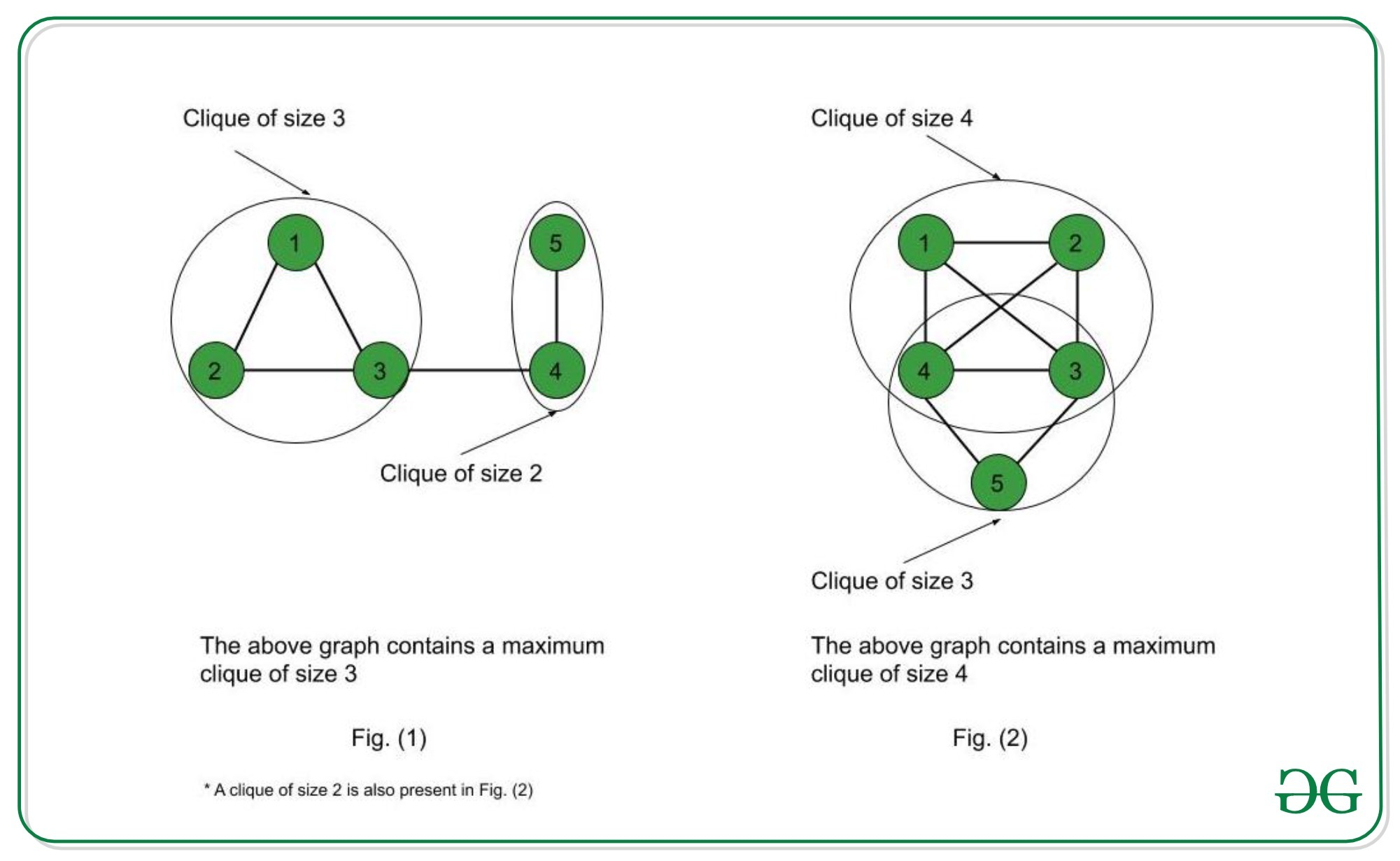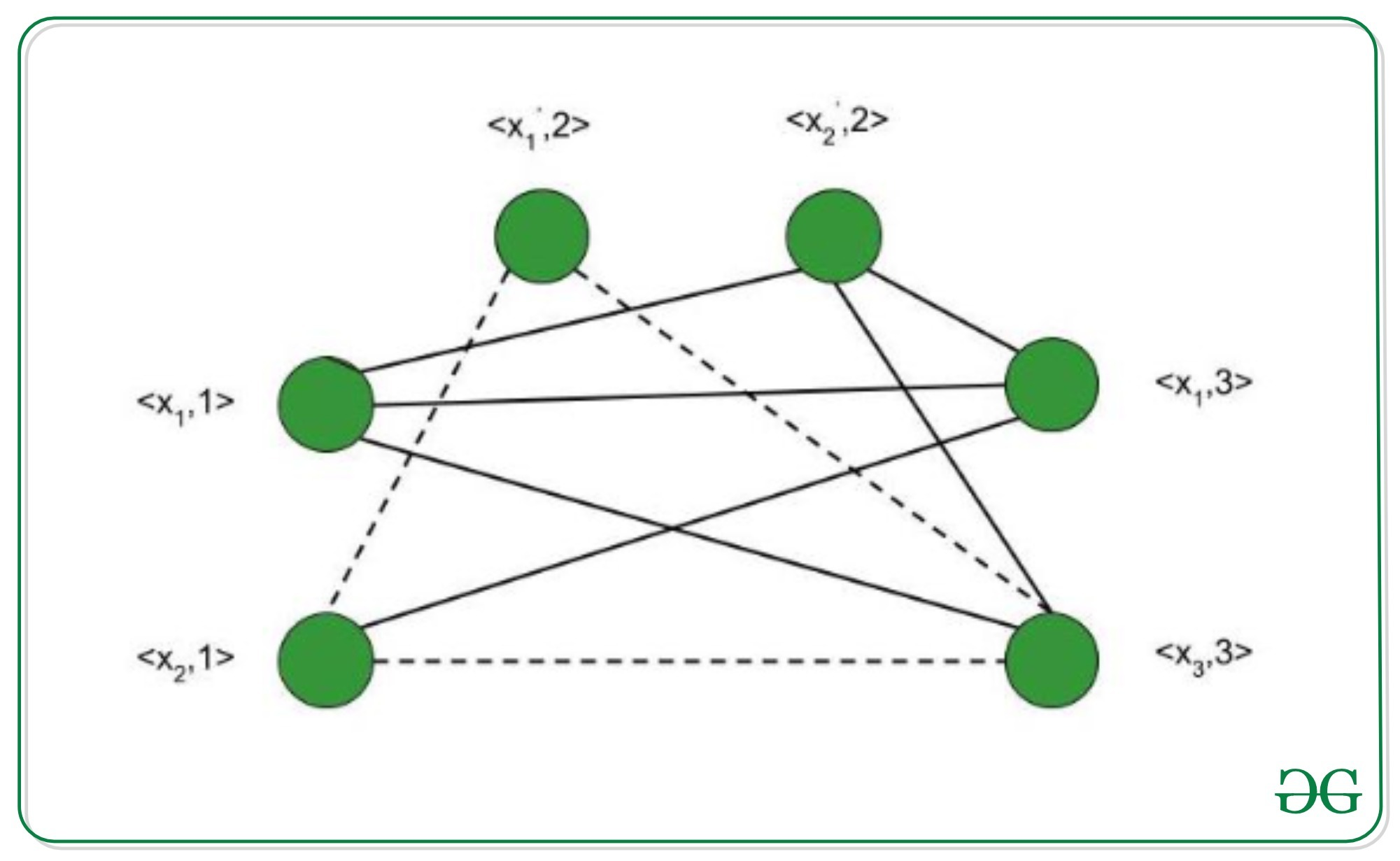Open in App
Not now

# Proof that Clique Decision problem is NP-Complete

• Difficulty Level : Hard
• Last Updated : 13 Jul, 2022

Prerequisite: NP-Completeness

A clique is a subgraph of a graph such that all the vertices in this subgraph are connected with each other that is the subgraph is a complete graph. The Maximal Clique Problem is to find the maximum sized clique of a given graph G, that is a complete graph which is a subgraph of G and contains the maximum number of vertices. This is an optimization problem. Correspondingly, the Clique Decision Problem is to find if a clique of size k exists in the given graph or not.To prove that a problem is NP-Complete, we have to show that it belongs to both NP and NP-Hard Classes. (Since NP-Complete problems are NP-Hard problems which also belong to NP)

The Clique Decision Problem belongs to NP – If a problem belongs to the NP class, then it should have polynomial-time verifiability, that is given a certificate, we should be able to verify in polynomial time if it is a solution to the problem.

Proof:

1. Certificate – Let the certificate be a set S consisting of nodes in the clique and S is a subgraph of G.
2. Verification – We have to check if there exists a clique of size k in the graph. Hence, verifying if number of nodes in S equals k, takes O(1) time. Verifying whether each vertex has an out-degree of (k-1) takes O(k2) time. (Since in a complete graph, each vertex is connected to every other vertex through an edge. Hence the total number of edges in a complete graph = kC2 = k*(k-1)/2 ). Therefore, to check if the graph formed by the k nodes in S is complete or not, it takes O(k2) = O(n2) time (since k<=n, where n is number of vertices in G).

Therefore, the Clique Decision Problem has polynomial time verifiability and hence belongs to the NP Class.

The Clique Decision Problem belongs to NP-Hard – A problem L belongs to NP-Hard if every NP problem is reducible to L in polynomial time. Now, let the Clique Decision Problem by C. To prove that C is NP-Hard, we take an already known NP-Hard problem, say S, and reduce it to C for a particular instance. If this reduction can be done in polynomial time, then C is also an NP-Hard problem. The Boolean Satisfiability Problem (S) is an NP-Complete problem as proved by the Cook’s theorem. Therefore, every problem in NP can be reduced to S in polynomial time. Thus, if S is reducible to C in polynomial time, every NP problem can be reduced to C in polynomial time, thereby proving C to be NP-Hard.

Proof that the Boolean Satisfiability problem reduces to the Clique Decision Problem
Let the boolean expression be – F = (x1 v x2) ^ (x1‘ v x2‘) ^ (x1 v x3)  where x1, x2, x3 are the variables, ‘^’ denotes logical ‘and’, ‘v’ denotes logical ‘or’ and x’ denotes the complement of x. Let the expression within each parentheses be a clause. Hence we have three clauses – C1, C2 and C3. Consider the vertices as – <x1, 1>; <x2, 1>; <x1’, 2>; <x2’, 2>; <x1, 3>; <x3, 3> where the second term in each vertex denotes the clause number they belong to. We connect these vertices such that –

1. No two vertices belonging to the same clause are connected.
2. No variable is connected to its complement.Thus, the graph G (V, E) is constructed such that – V = { <a, i> | a belongs to Ci } and E = { ( <a, i>, <b, j> ) | i is not equal to j ; b is not equal to a’ } Consider the subgraph of G with the vertices <x2, 1>; <x1’, 2>; <x3, 3>. It forms a clique of size 3 (Depicted by dotted line in above figure) . Corresponding to this, for the assignment – <x1, x2, x3> = <0, 1, 1>  F evaluates to true. Therefore, if we have k clauses in our satisfiability expression, we get a max clique of size k and for the corresponding assignment of values, the satisfiability expression evaluates to true. Hence, for a particular instance, the satisfiability problem is reduced to the clique decision problem.

Therefore, the Clique Decision Problem is NP-Hard.

Conclusion
The Clique Decision Problem is NP and NP-Hard. Therefore, the Clique decision problem is NP-Complete.